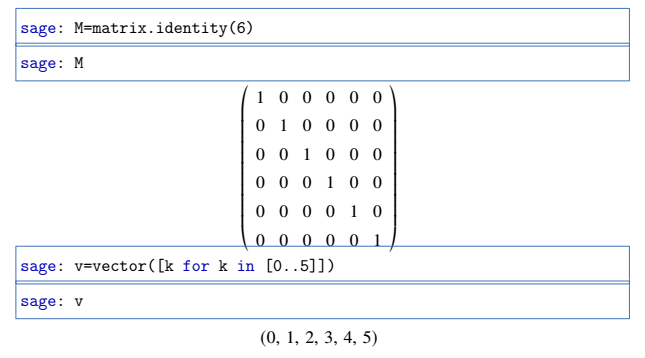# Revision history [back]

### SageTeX: sagecommandline problem

As a simple example of the problem I have encountered

\begin{sagecommandline}
sage: M=matrix.identity(6)
sage: M
sage: v=vector([k for k in [0..5]])
sage: v
\end{sagecommandline}


the output looks fine except that the printed matrix $M$ overlaps with the definition of the vector $v.$### SageTeX: sagecommandline problem

As a simple example of the problem I have encountered

\begin{sagecommandline}
sage: M=matrix.identity(6)
sage: M
sage: v=vector([k for k in [0..5]])
sage: v
\end{sagecommandline}


the output looks fine except that the printed matrix $M$ overlaps with the definition of the vector $v.$Is there a way to add some vertical space below the displayed matrix?